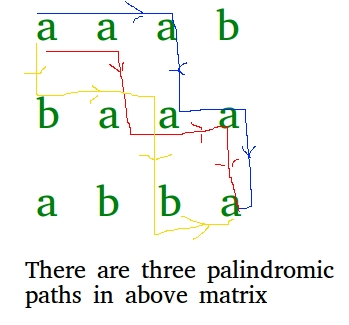# Number of palindromic paths in a matrix

• Difficulty Level : Hard
• Last Updated : 28 Jun, 2021

Given a matrix containing lower alphabetical characters only, we need to count number of palindromic paths in given matrix. A path is defined as a sequence of cells starting from top-left cell and ending at bottom-right cell. We are allowed to move to right and down only from current cell.

Examples:

```Input : mat[][] = {"aaab”,
"baaa”
“abba”}
Output : 3

Number of palindromic paths are 3 from top-left to
bottom-right.
aaaaaa (0, 0) -> (0, 1) -> (1, 1) -> (1, 2) ->
(1, 3) -> (2, 3)
aaaaaa (0, 0) -> (0, 1) -> (0, 2) -> (1, 2) ->
(1, 3) -> (2, 3)
abaaba (0, 0) -> (1, 0) -> (1, 1) -> (1, 2) ->
(2, 2) -> (2, 3)    ```We can solve this problem recursively, we start from two corners of a palindromic path(top-left and bottom right). In each recursive call, we maintain a state which will constitute two cells one from starting and one from end which should be equal for palindrome property. If at a state, both cell characters are equal then we call recursively with all possible movements in both directions.
As this can lead to solving same subproblem multiple times, we have taken a map memo in below code which stores the calculated result with key as indices of starting and ending cell so if subproblem with same starting and ending cell is called again, result will be returned by memo directly instead of recalculating again.
Please see below code for better understanding,

## CPP

 `// C++ program to get number of palindrome``// paths in matrix``#include ` `using` `namespace` `std;` `#define R 3``#define C 4` `// struct to represent state of recursion``// and key of map``struct` `cells``{``    ``//  indices of front cell``    ``int` `rs, cs;` `    ``//  indices of end cell``    ``int` `re, ce;``    ``cells(``int` `rs, ``int` `cs, ``int` `re, ``int` `ce)``        ``: rs(rs)``        ``, cs(cs)``        ``, re(re)``        ``, ce(ce)``    ``{``    ``}` `    ``// operator overloading to compare two``    ``// cells which rs needed for map``    ``bool` `operator<(``const` `cells& other) ``const``    ``{``        ``return` `((rs != other.rs) || (cs != other.cs)``                ``|| (re != other.re) || (ce != other.ce));``    ``}``};` `// recursive method to return number``//  of palindromic paths in matrix``// (rs, cs) ==> Indices of current cell``//              from a starting point (First Row)``// (re, ce) ==> Indices of current cell``//              from a ending point (Last Row)``// memo     ==> To store results of``//              already computed problems``int` `getPalindromicPathsRecur(``char` `mat[R][C],``                             ``int` `rs, ``int` `cs,``                             ``int` `re, ``int` `ce,``                             ``map& memo)``{``    ``// Base Case 1 : if any index rs out of boundary,``    ``// return 0``    ``if` `(rs < 0 || rs >= R || cs < 0 || cs >= C)``        ``return` `0;``    ``if` `(re < 0 || re < rs || ce < 0 || ce < cs)``        ``return` `0;` `    ``// Base case 2 : if values are not equal``    ``// then palindrome property rs not satisfied,``    ``// so return 0``    ``if` `(mat[rs][cs] != mat[re][ce])``        ``return` `0;` `    ``// If we reach here, then matrix cells are same.` `    ``// Base Case 3 : if indices are adjacent then``    ``// return 1``    ``if` `(``abs``((rs - re) + (cs - ce)) <= 1)``        ``return` `1;` `    ``//  if result rs precalculated, return from map``    ``if` `(memo.find(cells(rs, cs, re, ce))``        ``!= memo.end())``        ``return` `memo[cells(rs, cs, re, ce)];` `    ``int` `ret = 0; ``// Initialize result` `    ``// calling recursively for all possible movements``    ``ret += getPalindromicPathsRecur(mat, rs + 1,``                                    ``cs, re - 1,``                                    ``ce, memo);``    ``ret += getPalindromicPathsRecur(mat, rs + 1,``                                    ``cs, re,``                                    ``ce - 1, memo);``    ``ret += getPalindromicPathsRecur(mat, rs,``                                    ``cs + 1, re - 1,``                                    ``ce, memo);``    ``ret += getPalindromicPathsRecur(mat, rs,``                                    ``cs + 1, re,``                                    ``ce - 1, memo);` `    ``// storing the calculated result in map``    ``memo[cells(rs, cs, re, ce)] = ret;` `    ``return` `ret;``}` `//  method returns number of palindromic paths in matrix``int` `getPalindromicPaths(``char` `mat[R][C])``{``    ``map memo;``    ``return` `getPalindromicPathsRecur(mat, 0, 0, R - 1, C - 1,``                                    ``memo);``}` `//  Driver code``int` `main()``{``    ``char` `mat[R][C] = { ``'a'``, ``'a'``, ``'a'``,``                      ``'b'``, ``'b'``, ``'a'``,``                       ``'a'``, ``'a'``, ``'a'``,``                      ``'b'``, ``'b'``, ``'a'` `};``    ``printf``(``"%d"``, getPalindromicPaths(mat));` `    ``return` `0;``}`
Output
`3`

Time Complexity : O((R x C)2)

This article is contributed by Utkarsh Trivedi. If you like GeeksforGeeks and would like to contribute, you can also write an article using write.geeksforgeeks.org or mail your article to review-team@geeksforgeeks.org. See your article appearing on the GeeksforGeeks main page and help other Geeks.